• 重要性质 1、向量组B=(β1，β2，……，βm)能由向量组A=(α1，α2，……，αm)线性表示的...3、一个向量可由向量组中其余向量线性表示，前提是这个向量组线性相关。线性相关的向量组中并不是任一向量都可由其余向...
重要性质
1、向量组B=(β1，β2，……，βm)能由向量组A=(α1，α2，……，αm)线性表示的充要条件是：
矩阵A=(α1，α2，……，αm)的秩=矩阵(α1，α2，……，αm，B)的秩。
2、向量组B能由向量组A线性表示，则向量组B的秩不大于向量A的秩。反之不一定成立。
3、一个向量可由向量组中其余向量线性表示，前提是这个向量组线性相关。线性相关的向量组中并不是任一向量都可由其余向量线性表示；但当其余向量线性无关时，这个向量必可由其余向量线性表示。
4、零向量可由任一组向量线性表示。
5、向量组α1，α2，……，αm中每个向量都可由向量组本身线性表示。
展开全文• 索引 原问题 证明 原问题   向量组 [ β 1 β 2 ⋯ β s ] \left[ \begin{matrix} {{\beta }_{1}} & {{\beta }_{2}} & \cdots & {{\beta }_{s}} \\ \end{matrix} \right] [β1​​β2​​⋯​βs​​]可由线性无关...


索引
原问题证明

原问题
向量组

[

β

1

β

2

⋯

β

s

]

\left[ \begin{matrix} {{\beta }_{1}} & {{\beta }_{2}} & \cdots & {{\beta }_{s}} \\ \end{matrix} \right]

可由线性无关的向量组

[

α

1

α

2

⋯

α

r

]

\left[ \begin{matrix} {{\alpha }_{1}} & {{\alpha }_{2}} & \cdots & {{\alpha }_{r}} \\ \end{matrix} \right]

线性表示，即有

[

β

1

β

2

⋯

β

s

]

=

[

α

1

α

2

⋯

α

r

]

B

,

\left[ \begin{matrix} {{\beta }_{1}} & {{\beta }_{2}} & \cdots & {{\beta }_{s}} \\ \end{matrix} \right]=\left[ \begin{matrix} {{\alpha }_{1}} & {{\alpha }_{2}} & \cdots & {{\alpha }_{r}} \\ \end{matrix} \right]B,

则

r

a

n

k

{

[

β

1

β

2

⋯

β

s

]

}

=

r

a

n

k

(

B

)

.

rank\left\{ \left[ \begin{matrix} {{\beta }_{1}} & {{\beta }_{2}} & \cdots & {{\beta }_{s}} \\ \end{matrix} \right] \right\}=rank\left( B \right).

证明
引理1：矩阵的初等行变换和初等列变换不改变原矩阵的秩。定义1：对单位矩阵

I

I

进行一次初等变换后得到的矩阵称为初等矩阵。引理2：对矩阵进行一次初等行（列）变换相当于在原矩阵左（右）边乘一个相应的初等矩阵。引理3：初等矩阵的逆还是初等矩阵。
首先对矩阵

B

B

进行拆解。

B

B

是一个

r

×

s

r\times s

的矩阵。令

t

=

r

a

n

k

(

B

)

≤

min

⁡

{

r

,

s

}

t=rank\left( B \right)\le \min \left\{ r,s \right\}

，则

B

B

一定可以由矩阵

[

I

t

×

t

O

t

×

(

s

−

t

)

O

(

r

−

t

)

×

t

O

(

r

−

t

)

×

(

s

−

t

)

]

\left[ \begin{matrix} {{I}_{t\times t}} & {{O}_{t\times \left( s-t \right)}} \\ {{O}_{\left( r-t \right)\times t}} & {{O}_{\left( r-t \right)\times \left( s-t \right)}} \\ \end{matrix} \right]

经过有限的一系列初等行列变换得到，即存在初等矩阵

P

1

(

a

)

,

P

2

(

a

)

,

.

.

.

,

P

m

(

a

)

(

a

)

P_{1}^{\left( a \right)},\text{ }P_{2}^{\left( a \right)},...,P_{m\left( a \right)}^{\left( a \right)}

和

P

1

(

b

)

,

P

2

(

b

)

,

.

.

.

,

P

m

(

b

)

(

b

)

P_{1}^{\left( b \right)},\text{ }P_{2}^{\left( b \right)},...,P_{m\left( b \right)}^{\left( b \right)}

，使得

B

=

(

∑

i

m

(

a

)

P

i

(

a

)

)

[

I

t

×

t

O

t

×

(

s

−

t

)

O

(

r

−

t

)

×

t

O

(

r

−

t

)

×

(

s

−

t

)

]

(

∑

i

m

(

b

)

P

i

(

b

)

)

.

B=\left( \sum\limits_{i}^{m\left( a \right)}{P_{i}^{\left( a \right)}} \right)\left[ \begin{matrix} {{I}_{t\times t}} & {{O}_{t\times \left( s-t \right)}} \\ {{O}_{\left( r-t \right)\times t}} & {{O}_{\left( r-t \right)\times \left( s-t \right)}} \\ \end{matrix} \right]\left( \sum\limits_{i}^{m\left( b \right)}{P_{i}^{\left( b \right)}} \right).

所以我们得到

[

β

1

β

2

⋯

β

s

]

=

[

α

1

α

2

⋯

α

r

]

{

∑

i

m

(

a

)

P

i

(

a

)

[

I

t

×

t

O

t

×

(

s

−

t

)

O

(

r

−

t

)

×

t

O

(

r

−

t

)

×

(

s

−

t

)

]

∑

i

m

(

b

)

P

i

(

b

)

}

\left[ \begin{matrix} {{\beta }_{1}} & {{\beta }_{2}} & \cdots & {{\beta }_{s}} \\ \end{matrix} \right]=\left[ \begin{matrix} {{\alpha }_{1}} & {{\alpha }_{2}} & \cdots & {{\alpha }_{r}} \\ \end{matrix} \right]\left\{ \sum\limits_{i}^{m\left( a \right)}{P_{i}^{\left( a \right)}}\left[ \begin{matrix} {{I}_{t\times t}} & {{O}_{t\times \left( s-t \right)}} \\ {{O}_{\left( r-t \right)\times t}} & {{O}_{\left( r-t \right)\times \left( s-t \right)}} \\ \end{matrix} \right]\sum\limits_{i}^{m\left( b \right)}{P_{i}^{\left( b \right)}} \right\}

⇒

[

β

1

β

2

⋯

β

s

]

⋅

(

∑

i

m

(

b

)

P

i

(

b

)

)

−

1

=

{

[

α

1

α

2

⋯

α

r

]

⋅

∑

i

m

(

a

)

P

i

(

a

)

}

[

I

t

×

t

O

t

×

(

s

−

t

)

O

(

r

−

t

)

×

t

O

(

r

−

t

)

×

(

s

−

t

)

]

.

\Rightarrow \left[ \begin{matrix} {{\beta }_{1}} & {{\beta }_{2}} & \cdots & {{\beta }_{s}} \\ \end{matrix} \right]\centerdot {{\left( \sum\limits_{i}^{m\left( b \right)}{P_{i}^{\left( b \right)}} \right)}^{-1}}=\left\{ \left[ \begin{matrix} {{\alpha }_{1}} & {{\alpha }_{2}} & \cdots & {{\alpha }_{r}} \\ \end{matrix} \right]\centerdot \sum\limits_{i}^{m\left( a \right)}{P_{i}^{\left( a \right)}} \right\}\left[ \begin{matrix} {{I}_{t\times t}} & {{O}_{t\times \left( s-t \right)}} \\ {{O}_{\left( r-t \right)\times t}} & {{O}_{\left( r-t \right)\times \left( s-t \right)}} \\ \end{matrix} \right].

记

[

β

1

(

1

)

β

2

(

1

)

⋯

β

s

(

1

)

]

=

[

β

1

β

2

⋯

β

s

]

(

∑

i

m

(

b

)

P

i

(

b

)

)

−

1

,

\left[ {{\begin{matrix} {{\beta }_{1}}^{\left( 1 \right)} & {{\beta }_{2}}^{\left( 1 \right)} & \cdots & {{\beta }_{s}} \\ \end{matrix}}^{\left( 1 \right)}} \right]=\left[ \begin{matrix} {{\beta }_{1}} & {{\beta }_{2}} & \cdots & {{\beta }_{s}} \\ \end{matrix} \right]{{\left( \sum\limits_{i}^{m\left( b \right)}{P_{i}^{\left( b \right)}} \right)}^{-1}},

[

α

1

(

1

)

α

2

(

1

)

⋯

α

r

(

1

)

]

=

[

α

1

α

2

⋯

α

r

]

∑

i

m

(

a

)

P

i

(

a

)

,

\left[ \begin{matrix} {{\alpha }_{1}}^{\left( 1 \right)} & {{\alpha }_{2}}^{\left( 1 \right)} & \cdots & {{\alpha }_{r}}^{\left( 1 \right)} \\ \end{matrix} \right]=\left[ \begin{matrix} {{\alpha }_{1}} & {{\alpha }_{2}} & \cdots & {{\alpha }_{r}} \\ \end{matrix} \right]\sum\limits_{i}^{m\left( a \right)}{P_{i}^{\left( a \right)}},

则可简写成

[

β

1

(

1

)

β

2

(

1

)

⋯

β

s

(

1

)

]

=

[

α

1

(

1

)

α

2

(

1

)

⋯

α

r

(

1

)

]

[

I

t

×

t

O

t

×

(

s

−

t

)

O

(

r

−

t

)

×

t

O

(

r

−

t

)

×

(

s

−

t

)

]

=

[

α

1

(

1

)

,

α

2

(

1

)

,

.

.

.

,

α

t

(

1

)

]

(

t

≤

r

)

\begin{aligned} & \left[ {{\begin{matrix} {{\beta }_{1}}^{\left( 1 \right)} & {{\beta }_{2}}^{\left( 1 \right)} & \cdots & {{\beta }_{s}} \\ \end{matrix}}^{\left( 1 \right)}} \right]=\left[ \begin{matrix} {{\alpha }_{1}}^{\left( 1 \right)} & {{\alpha }_{2}}^{\left( 1 \right)} & \cdots & {{\alpha }_{r}}^{\left( 1 \right)} \\ \end{matrix} \right]\left[ \begin{matrix} {{I}_{t\times t}} & {{O}_{t\times \left( s-t \right)}} \\ {{O}_{\left( r-t \right)\times t}} & {{O}_{\left( r-t \right)\times \left( s-t \right)}} \\ \end{matrix} \right] \\ & \text{ }=\left[ \alpha _{1}^{\left( 1 \right)},\alpha _{2}^{\left( 1 \right)},...,\alpha _{t}^{\left( 1 \right)} \right]\text{ }\left( t\le r \right) \\ \end{aligned}

下面分步骤进行分析。

[

β

1

(

1

)

β

2

(

1

)

⋯

β

s

(

1

)

]

\left[ {{\begin{matrix} {{\beta }_{1}}^{\left( 1 \right)} & {{\beta }_{2}}^{\left( 1 \right)} & \cdots & {{\beta }_{s}} \\ \end{matrix}}^{\left( 1 \right)}} \right]

是由

[

β

1

β

2

⋯

β

s

]

\left[ \begin{matrix} {{\beta }_{1}} & {{\beta }_{2}} & \cdots & {{\beta }_{s}} \\ \end{matrix} \right]

右乘

(

∑

i

m

(

b

)

P

i

(

b

)

)

−

1

{{\left( \sum\limits_{i}^{m\left( b \right)}{P_{i}^{\left( b \right)}} \right)}^{-1}}

得到，即右乘一系列初等矩阵

(

P

m

(

b

)

(

b

)

)

−

1

,

(

P

m

(

b

)

−

1

(

b

)

)

−

1

,

.

.

.

,

(

P

1

(

b

)

)

−

1

{{\left( P_{m\left( b \right)}^{\left( b \right)} \right)}^{-1}},{{\left( P_{m\left( b \right)-1}^{\left( b \right)} \right)}^{-1}},...,{{\left( P_{1}^{\left( b \right)} \right)}^{-1}}

得到，所以有

r

a

n

k

{

[

β

1

β

2

⋯

β

s

]

}

=

r

a

n

k

{

[

β

1

(

1

)

β

2

(

1

)

⋯

β

s

(

1

)

]

}

rank\left\{ \left[ \begin{matrix} {{\beta }_{1}} & {{\beta }_{2}} & \cdots & {{\beta }_{s}} \\ \end{matrix} \right] \right\}=rank\left\{ \left[ {{\begin{matrix} {{\beta }_{1}}^{\left( 1 \right)} & {{\beta }_{2}}^{\left( 1 \right)} & \cdots & {{\beta }_{s}} \\ \end{matrix}}^{\left( 1 \right)}} \right] \right\}

[

α

1

(

1

)

α

2

(

1

)

⋯

α

r

(

1

)

]

\left[ \begin{matrix} {{\alpha }_{1}}^{\left( 1 \right)} & {{\alpha }_{2}}^{\left( 1 \right)} & \cdots & {{\alpha }_{r}}^{\left( 1 \right)} \\ \end{matrix} \right]

是由

[

α

1

α

2

⋯

α

r

]

\left[ \begin{matrix} {{\alpha }_{1}} & {{\alpha }_{2}} & \cdots & {{\alpha }_{r}} \\ \end{matrix} \right]

右乘一系列初等矩阵

∑

i

m

(

a

)

P

i

(

a

)

\sum\limits_{i}^{m\left( a \right)}{P_{i}^{\left( a \right)}}

得到，有

r

a

n

k

{

[

α

1

(

1

)

α

2

(

1

)

⋯

α

r

(

1

)

]

}

=

r

a

n

k

{

[

α

1

α

2

⋯

α

r

]

}

=

r

rank\left\{ \left[ {{\begin{matrix} {{\alpha }_{1}}^{\left( 1 \right)} & {{\alpha }_{2}}^{\left( 1 \right)} & \cdots & {{\alpha }_{r}} \\ \end{matrix}}^{\left( 1 \right)}} \right] \right\}=rank\left\{ \left[ \begin{matrix} {{\alpha }_{1}} & {{\alpha }_{2}} & \cdots & {{\alpha }_{r}} \\ \end{matrix} \right] \right\}=r

在步骤2已经知道

[

α

1

(

1

)

α

2

(

1

)

⋯

α

r

(

1

)

]

\left[ {{\begin{matrix} {{\alpha }_{1}}^{\left( 1 \right)} & {{\alpha }_{2}}^{\left( 1 \right)} & \cdots & {{\alpha }_{r}} \\ \end{matrix}}^{\left( 1 \right)}} \right]

也是一个极大线性无关组的基础上，结合上面得到的

[

β

1

(

1

)

β

2

(

1

)

⋯

β

s

(

1

)

]

=

[

α

1

(

1

)

,

α

2

(

1

)

,

.

.

.

,

α

t

(

1

)

]

\left[ {{\begin{matrix} {{\beta }_{1}}^{\left( 1 \right)} & {{\beta }_{2}}^{\left( 1 \right)} & \cdots & {{\beta }_{s}} \\ \end{matrix}}^{\left( 1 \right)}} \right]=\left[ \alpha _{1}^{\left( 1 \right)},\alpha _{2}^{\left( 1 \right)},...,\alpha _{t}^{\left( 1 \right)} \right]

和

t

≤

r

t\le r

的条件，显然有

r

a

n

k

{

[

β

1

(

1

)

β

2

(

1

)

⋯

β

s

(

1

)

]

}

=

r

a

n

k

{

[

α

1

(

1

)

,

α

2

(

1

)

,

.

.

.

,

α

t

(

1

)

]

}

=

t

.

rank\left\{ \left[ {{\begin{matrix} {{\beta }_{1}}^{\left( 1 \right)} & {{\beta }_{2}}^{\left( 1 \right)} & \cdots & {{\beta }_{s}} \\ \end{matrix}}^{\left( 1 \right)}} \right] \right\}=rank\left\{ \left[ \alpha _{1}^{\left( 1 \right)},\alpha _{2}^{\left( 1 \right)},...,\alpha _{t}^{\left( 1 \right)} \right] \right\}=t.

综上即可证得

r

a

n

k

{

[

β

1

β

2

⋯

β

s

]

}

=

t

=

r

a

n

k

(

B

)

rank\left\{ \left[ \begin{matrix} {{\beta }_{1}} & {{\beta }_{2}} & \cdots & {{\beta }_{s}} \\ \end{matrix} \right] \right\}=t=rank\left( B \right)


展开全文• 线性代数之线性相关线性表示的求法 线性相关 向量是n个m维(每个向量分量的个数)的向量，若存在一不全为0的 使得 则 是线性相关的，反之线性无关。 线性无关即等价于以下命题： 线性不相关 找不到一不全0...
线性代数之线性相关线性表示的求法
线性相关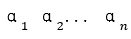向量是n个m维(每个向量分量的个数)的向量，若存在一组不全为0的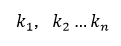使得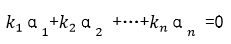则是线性相关的，反之线性无关。
线性无关即等价于以下命题：
线性不相关找不到一组不全0的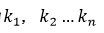使得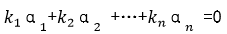全为0
几种情况:
关于单个向量
向量组中两个向量成比例，则两个向量必线性相关含零向量的任向量组必线性相关(取0向量的系数为1或者k，其余均为0)一个零向量必线性相关一个非零向量必然线性无关一个向量线性相关的充要条件是向量为0向量
线性表示
如果向量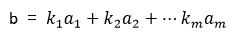则b是向量组A的线性组合，这是向量b可有向量组A线性表示。这里其实转换为了方程有解，全是0也是有解。
特别的：
线性表示时系数可以全是00向量可有任意向量组表示。
任何向量都可由 (1,0,...0),(0,1,0...0),(0,0,1...0) ...(0,0...0...1)表示

线性相关例子汇总
判断线性相关（不含参数）
该方法是根据矩阵的秩的定义来求，如果找到k阶子式为0，而k-1阶不为0，那么k-1即该矩阵的秩。
#Sample1（示例一）,判断如下向量组是否线性相关：
1：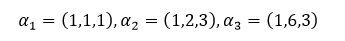2：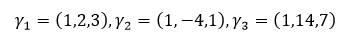解：针对第一题：
Step1：首先我们先立方程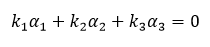针对其解的情况来判断向量组是否线性相关(有解)或者无关（无解）。
Step2：于是我们得到下式：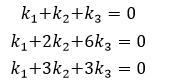Step3： 我们对k的行列式化简得到如下行列式：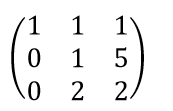该行列式不为0，所以当前关于k的方程组有唯一解，即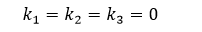所以当前向量组里的向量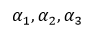线性无关。
针对第二题：同样的思路
Step1：设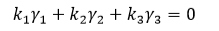Step2：于是我们得到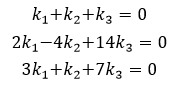Step3：针对k化简得到如下行列式，易得其为0,所以k有非零解。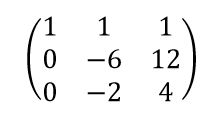Step4：因为关于k的解有无穷个，所有这里取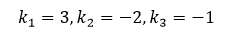换言之存在不全为0的数使得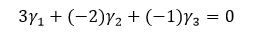即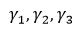线性相关。
判断线性相关(含参数)
针对这种类型的问题，一般将它们按照列(行)的形式构成矩阵，对矩阵做行(列)变换，使矩阵变成阶梯型。最后根据矩阵中参数的取值是否使得其所在行（列）为零行来判断向量组的线性相关性。（参数所在行全为0则行列式为0，线性无关，否则相关）。
#Sample2（示例二）：已知向量组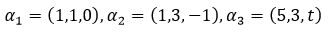判断其相关性。
解：
Step1：因这里向量组的向量个数和向量的维数相同，所以可以按照列组成行列式。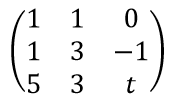Step2：第1行的-1倍加到第2行上去，第1行的-5倍加到第3行上去，则得: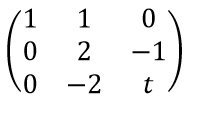即行列式等于2(t-1)
Step3:针对Step2里的t进行讨论，如果t=1，则行列式等于0(即方程有无穷非非零解)，则线性相关，如果t≠1则行列式不等于0（即方程只有零解），则线性无关。
线性表示例子汇总
阶梯法判断线性表示
利用矩阵的初等变换不改变矩阵的列的线性关系的特点求解。
#Sample3（示例三）
向量β=（4,4,1，2）是否可由如下向量组线性表示，如果可以，写出表达式。
1: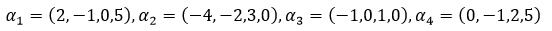2: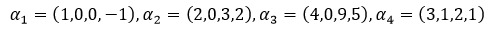解：
针对第一题：
Step1：用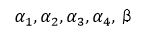作为列向量构成矩阵A，则A为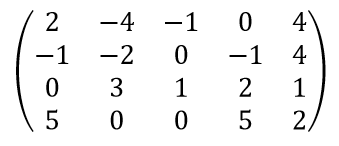Step2：交换第1和第2行，则化为：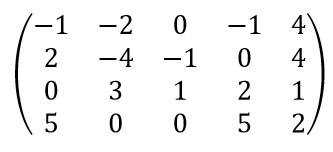Step3：第1行的2倍加到第2行上去，第1行的5倍加到第4行上去，第1行乘-1，则最终化为：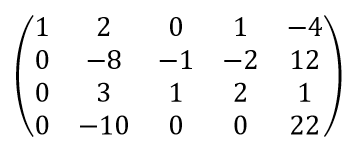Step4：在对step3里的矩阵化简，第3行的3倍加到第2、4行上去，则得：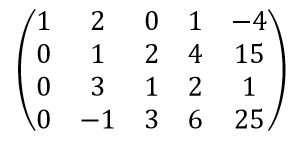Step5：在对step4里的矩阵化简，第2行的-3倍加到第3行上去，第2行的1倍加到第3行上去，则得：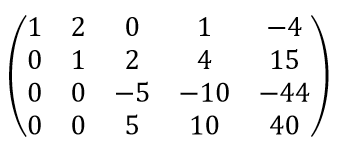Step6：在对step5里的矩阵化简，第3行的1倍加到第4行上去，第3行除以-5，则得：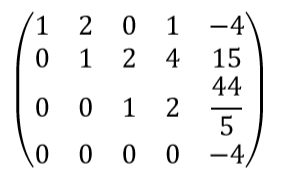Step7：由A的阶梯型可知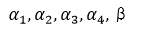这5个向量的向量组的秩（阶梯型里非零行的行数）是4，所以该向量组的秩必定包含β，即β不能由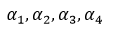线性表示。

针对第二题：
类似第一题，可将构成的矩阵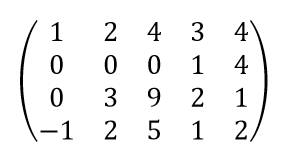化简为：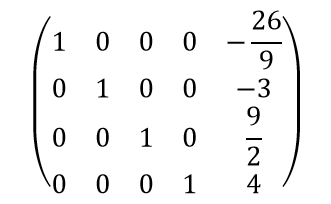则可见即β可由线性表示，即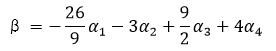展开全文• 一、当向量组 个数大于维数时 ????个????维向量组成的向量组,当?...时向量组线性相关 ...(向量个数）,故向量组线性...当向量个数等于向量维数时,向量组线性相关的充要条件是该向量组构成的矩阵????的行列式????=0 而向..
一、当向量组 个数大于维数时
𝑚个𝑛维向量组成的向量组,当𝑛<𝑚时向量组线性相关
对应矩阵𝑟(𝐴)≤𝑚𝑖𝑛(𝑛,𝑚),由𝑛<𝑚,则𝑟(𝐴)<𝑚(向量个数）,故向量组线性相关.维数小于个数,线性相关;维数大于个数,不一定（可能线性相关也可能线性无关）
二、当向量组 个数等于维数时（通过行列式判断）
当向量个数等于向量维数时,向量组线性相关的充要条件是该向量组构成的矩阵𝐴的行列式𝐴=0
而向量组线性无关的充要条件是𝐴≠0
三、当向量组 个数小于维数时（通过秩判断）
当向量组的个数小于维数时候，设列向量组𝐴:𝛼1,𝛼2,...,𝛼m构成矩阵𝐴=𝛼1,𝛼2,...,𝛼𝑚,则向量组𝐴线性相关的充要条件是矩阵𝐴的秩小于向量个数𝑚,即𝑟(𝐴)<𝑚;向量组𝐴线性无关的充要条件是矩阵𝐴的秩等于向量个数𝑚,即𝑟(𝐴)=𝑚.

展开全文• 如果向量组α，β，γ线性无关<=>矩阵A=(αT，βT，γT)满秩<=>|A|≠0
• 第一节 向量组线性相关性   一．数学概念 定义1.1 n个有次序的数 ，所组成的数组称为n维向量，这n个数称为该向量的n个分量，第i个数 称为第i个分量。 定义1. 2 给定向量组A： ，对于任何一组实数 ，...
• 第三节 向量空间 一．数字概念 定义3.1 设V是n维向量集合，且非空，若 (i) 则, ； (ii) 则 。...定义3.2 设 是两个向量...(ii) V中的任一向量都可由 线性表示，则称向量组 是向量空间V的一个基，r称为向量向量空间
• 向量组及其线性组合 向量组线性相关性 向量组的秩 向量空间 线性方程组解的结构 向量组及其线性组合 nn个有次序的数a1,a2,...,ana_1,a_2,...,a_n所组成的一个有序数组(a1,a2,...,an)(a_1,a_2,...,a_n)...
• 向量和向量组 以下讨论同样适用于行矩阵： 列矩阵的每一个元素被看作空间内的一个向量...当向量组B的所有向量bi都能用A线性表示时，称向量组B能被向量组A先行表示。这个关系不一定可逆。 当向量方程组AX=0有非0解...
• 参考：《线性代数》同济大学第四版 1. 向量组及其线性组合  1）向量：定义；实向量、复向量；行向量、列向量； ... 2）向量空间：定义；... 4）定理1：向量能由向量组线性表示的充要条件  5）定理2
• 向量组线性相关，线性无关(非常重要) 线性相关 满足上式且k是一组不全为0的数，则称α1，…αn线性相关。 向量组α1，…αn线性相关的充要条件 至少存在一个向量αi可以由其他的向量线性表出 矩阵A=(α1，…αn...
• 定义4：给定向量组，如果存在不全为零的数，使得，则称向量...定理4:向量组线性相关的充分必要条件是它所构成的矩阵的秩小于向量个数m； 向量组线性无关的充分必要条件是 特别,当m=n,即向量组中向量个数等于向量的维
• 机器学习的数学基础 线性代数 文章目录机器学习的数学基础线性代数线性代数行列式1.行列式按行（列）展开定理矩阵...有关向量组线性表示2.有关向量组的线性相关性3.有关向量组线性表示4.向量组的秩与矩阵的秩之...机器学习 人工智能 算法
• 若干个同维数的列向量（或同维数的行向量）所组成的集合叫做向量组 **线性相关性** 几个定义 线性组合的系数 给定向量组 A：a1，a2，…，am，对于任何一组实数 k1，k2，…，km，表达式 k1a1+k2a2+…+km...
• ２、[定理１]向量b能由向量组A线性表示的充要条件是R(A)=R(A,b)。 ３、若A与B列等价，则A的列向量组与B的列向量组等价；若A与B行等价，则A的行向量组与B的行向量组等价。 ４、[定理２]向量组B能由向量组
• 第四章 n维向量线性方程编者注：第四章为n维向量线性方程，在线性代数整个课程学习中的地位都是至关重要的。线性代数作为工科生的一门基础学科，对于以后个人的发展都是有着极大的促进作用的。这一章小助手...高等数学
• n维向量组 注意点 任意一个n维向量都可以由n维基本单位向量表示 0向量时任意向量组的线性组合 向量组A中任意一个向量都可以由这个向量组表示 ...向量组线性相关的充要条件是其中至少一个向量可以由
• n维列向量组α1,α2,...,αm,m, \alpha_2,...,\alpha_m, m 线性无关，则n维列向量组β1,β2,...,βm\beta_1,\beta_2,...,\beta_m线性无关的充要条件是（D） A. 向量组α1,α2,...,αm\alpha_1, \alpha_2,...,\alpha...
• 矩阵A的列向量组可以由矩阵B的列向量组线性表示时 一定存在C有A=BC,（你把每个表达式写出来,组合一下就可以得到这个式子） R（A)=R(BC) 又因为R(A)=R(BC)<= min{R（C）,R(B)}<=R(B） ...
• 原来相关，增加一个向量后，向量组还是线性相关，只不过它不一定能被其余向量线性表示。原来无关减少一个向量后，还是无关。原来相关减少一个向量后，若减少的是那个唯一能被其余向量线性表示的向量...机器学习 算法 matlab 设计模式
• 定义1：n个有次序的数 a1,a2,....an所组成的...n维向量表示方法 n维向量写成一行，称为行向量，也就是行矩阵，如： n维向量写成一列，称为列向量，也就是列矩阵，如： 注意: 1.行向量和列向量都按照矩阵
• 线性相关的充分必要条件向量组中至少有一个向量可由其余向量线性表示 线性表示 定义 指线性空间中的一个元素可通过另一组元素的线性运算来表示。零向量可由任一组向量线性表示。 等价向量组 性质 ...
• 1向量组及其线性组合 n维向量——n个有次序的苏a1，a2，a3，am所组成的数组成为n维向量， n维向量写出一行成为行向量，组成一列成为列向量 a=(a1a2⋮an) a= \left( \begin{matrix} a1\\ a2\\ \vdots\\ a_n \end{...
• 文章目录第三讲 向量组线性相关和线性无关的几何意义线性变换的几何意义向量及向量组的线性相关性判别线性相关性的七大定理 第三讲 向量组 本章四大问题：线性表出、线性相关、极大线性无关组、等价向量组 矩阵...
• 1、n个有次序的数，组成的数组称为n维向量，这n个数称作分量，第i个数称作第i个分量。...3、向量组B可以由向量组A线性表示的充要条件是R(A)=R(A,B)，而两个向量组等价的条件是R(A)=R(B) =R(A,B) 4、线性相关...
• 文章目录A 线性表示B 基与维数C 向量的坐标D 过渡矩阵 A 线性表示 <1> 定义1（线性表示） <2> 定义2（线性相关） <3> 定义3（线性无关） 注： {α1,α2...αn}\{\alpha_1,\alpha_2...\alpha_...
• 1.1.1 二元线性方程与二阶行列式 1.1.2 三阶行列式 1.2 全排列和对换 1.2.1 排列及其逆序数 全排列：把n个不同的元素排成一列 所有排列的种数： 标准排列：对于n个不同的元素，先......